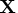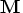Previous Page | Next Page

 Language Reference

## Subscripts:   [ ]

select submatrices

matrix[rows,columns]
matrix[elements]

Subscripts are used with matrices to select submatrices, where rows and columns are expressions that evaluate to scalars or vectors. If these expressions are numeric, they contain valid subscript values of rows and columns in the argument matrix. If a row or column expression is a character matrix, then it refers to columns or rows in the argument matrix assigned corresponding labels by a MATTRIB statement or READ statement. A subscripted matrix can appear on the left side of the equal sign. The dimensions of the target submatrix must conform to the dimensions of the source matrix. See the section "Using Matrix Expressions" for further information.

For example, the following statements select the element in the second row and third column of, producing the matrix:

```
x={1 2 3,
4 5 6,
7 8 9};
a=3;
m=x[2,a];
```
Here is the matrix:
```
M             1 row      1 col    (numeric)

6
```
The following statements select row 2 and columns 1 through 3 of, producing the matrix:
```
a=1:3;
m=x[2,a];
```
Here is the matrix:
```
M             1 row      3 cols    (numeric)

4         5         6
```
The following statements select the element in the second row and third column of, producing the matrix:
```
x={1 2 3,
4 5 6,
7 8 9};
MATTRIB x colname = {'col1' 'col2' 'col3'}
rowname = {'row1' 'row2' 'row3'};
a='col3';
m=x['row2',a];
```
Here is the matrix:
```
M             1 row      1 col    (numeric)

6
```Previous Page | Next Page | Top of Page